# Fourier Analysis and the Significance of Odd and Even Functions

## Homework Statement

Q1. a) In relation to Fourier analysis state the meaning and significance of
4
i) odd and even functions ii) half-wave symmetry {i.e. f(t+π)= −f(t)}.
Illustrate each answer with a suitable waveform sketch.
b) State by inspection (i.e. without performing any formal analysis) all you can about each of the periodic waveforms shown in FIGURE 1 in terms of their Fourier series when analysed about t = 0.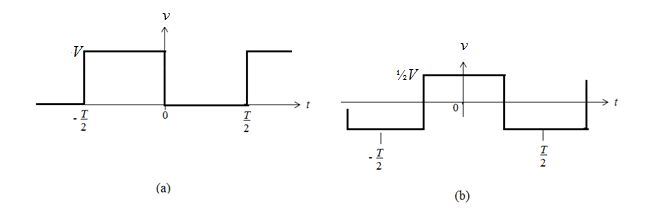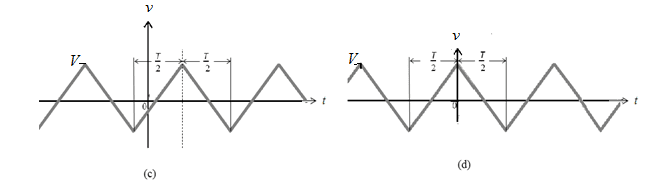## Homework Equations

No equations required ( I don't think)

## The Attempt at a Solution

So I have attached my rough answers for 1a) i/ii.
For 1b) im just have trouble getting a head start. I don't know how much depth that want for it or even if there is much to say?

Would i just go about explaining if they are even/odd functions which would tell us something about the symmetry & what sine/cosine terms they have or if they are neither. Any x/y axis shifts e.g. in (c) looks like it's been shifted forwards compared to (d). I just want to get a rough idea of what im writing.

So for example for (a) Would i just have to say it's an odd function. Thus meaning it would have symmetry about the origin. For this function it would only consist of sine terms. Other then this how much further do i take it?

#### Attachments

FactChecker
Gold Member
So for example for (a) Would i just have to say it's an odd function. Thus meaning it would have symmetry about the origin. For this function it would only consist of sine terms. Other then this how much further do i take it?
But it's not exactly an odd function: f(-x) does not equal -f(x). Is there something you can do to relate it to an odd function? What would that imply for the fourier series?

But it's not exactly an odd function: f(-x) does not equal -f(x). Is there something you can do to relate it to an odd function? What would that imply for the fourier series?
Oh yeah! So by shifting it to the right by T/2 you would get a regular square wave? and thus making it have only sine terms. With regards to to whether they have symmetry I actually cannot tell now, it doesn't look symmetrial about the y axis so it's not an even function but it doesn't have symmetry about the origin unless it was shifted left on the t-axis by -T/4. Am i correct in thinking that? I just don't know what a blanket answer per waveform would be and how much information is required when it states no formal analysis. Which to me would be no mathmatical solving thus not finding a0, an or bn.How did my write up for the definitions of 1a) i/ii look?

FactChecker
Gold Member
Shifting it that way will not make it either even or odd and will not help. Shifting it down is what is needed. Y-V/2 is an odd function. What does that mean in terms of the Fourier series for Y?

Last edited:
Okay think i got it now :) thanks for your help.

@Connorm1

Hi all, having a look at this now and I am on the same boat as you were - I don't know how much detail they want and where to start with part (b). I see that you were trying to move the wave down the Y-axis to make it Odd, why not shift it to the right by T/4 so that it creates an even wave?

Also if you have had feedback re. this question were you successful / what was the general 'jist' were they looking for?

FactChecker
Gold Member
To make an even function, you could shift it down by V/2 and then right by T/4, but I don't think that helps much. The problem question was to describe the original coefficients. The shift down only changes the constant coefficient, so you can easily say that the coefficients of the cosines are zero.

Ah I see what you mean I think, so I could maybe say the following:
- The wave is neither odd nor even
- a0 =0 & an = 0

Surely that can't be all there is?

FactChecker
Gold Member
##a_0 = V/2; a_n = 0##. Without analysis, I don't know how much more you can say. You can probably say that ##b_1 > 0##. The might be more. I am not an expert and I don't know how much they are supposed to know.

•Jason-Li
@FactChecker Thanks a lot for the insight, not sure how I messed up the A0 figure. I have one query regarding a similar waveform in the learning materials that has been 'shifted odd' and I am unsure how this wave is 'odd' and not neither? wondering if you can give some insight. Thanks again.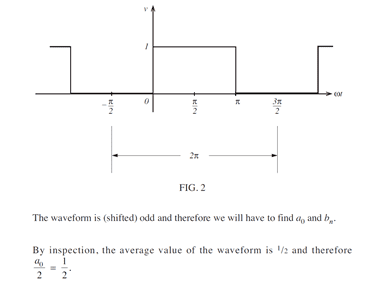#### Attachments

FactChecker
Gold Member
The function drawn is not odd, but it is the result of shifting an odd function up by 1/2.

I thought it wasn't odd, the learning materials seem to be incorrect, it is implying that the diagram shown is odd - seems to be a recurring theme with this learning materials!

bobob
Gold Member
The significance of odd and even functions is that if a function is even, all of the sine coefficients are zero and if a function is odd, then all of the cosine coefficients are zero. Odd functions have no constant offset (a_0/2) term. Even functions which are not symmetric about the horizontal axis will have a non-zero constant (DC offset).

So, if it's possible to shift your function to make the function purely even or purely odd, it's much easier to shift the function and obtain the expansion and shift it back (if neccessary). Generally, what matters most is the harmonic content, not the choices of coordinate axes. Shifting the coordinates does not affect anything but the way the solution "looks" aside from a possible constnt offset. It doesn't change the relative magnitude of the harmonic contributions.

In your figures, (a) is neither, (b) is even, (c) is neither, (d) is even, (e) and (f) are even. You can make any of those functions odd by shifting the coordinates (the same way you can shift the cosine by pi/2 to get a sine. The whole point here (at least according those who beat it into me as a student) is to exploit symmetry to make things simpler and get the physically meanigful information, rather than brute force a mess without any intuition.

•Jason-Li
Hi @bobob thanks for the help that really clears things up! I have tried bulking out the answer for each as follows, does that look correct to you? ( I realise this just builds on what you wrote )

a) Function is neither odd nor even.
a0= V/2, an=0

b) Function is even hence will only contain cosine terms and potentially one constant term with no sine terms.
a0 = 0, bn = 0

c) Function is neither odd nor even.
a0 = 0
It has half-wave symmetry.

d) Function is even hence will only contain cosine terms and potentially one constant term with no sine terms.
The wave is half symmetric.
a0 = 0, bn = 0

e) Function is even hence will only contain cosine terms and potentially one constant term with no sine terms.
a0 = 0, bn = 0
It has half-wave symmetry.

f) Function is even when inspected between -8t & 8t hence will only contain cosine terms and potentially one constant term with no sine terms.
a0 = 0, bn = 0

FactChecker
Gold Member
I thought it wasn't odd, the learning materials seem to be incorrect, it is implying that the diagram shown is odd - seems to be a recurring theme with this learning materials!
It is only poorly worded. You can take an odd function and shift it up to get the function graphed in the diagram. That function in the diagram is no longer odd. But its coefficients are identical to the original odd function except for ##a_0##.

@FactChecker Yeah in the materials the first graph is even (FIG.1) and the second graph (FIG.2) which is FIG.1 'shifted' is now odd, however I would say that it is neither odd nor even, unless as you say it is shifted down the 'Y' axis. There is however no mention of this Y-axis shift in these examples.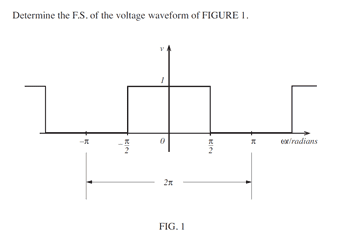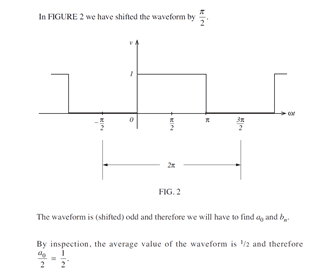FactChecker
•